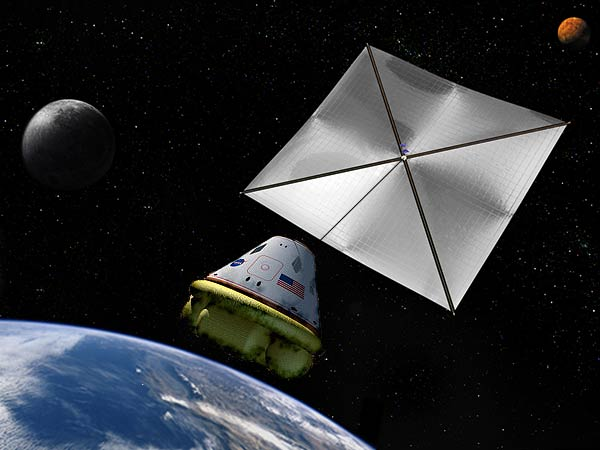# Solar Powered SpaceshipThe sun puts out roughly $4 \times 10^{26}$ Watts of electromagnetic energy. The sun emits as a blackbody at roughly 6000 K. The peak wavelength of the solar emission, i.e. the wavelength at which the intensity is highest, is approximately 500 nm (which is green). For the purposes of this discussion, let's assume all of the sun's radiation comes out at this wavelength. If we think about the radiation as photons, each photon carries a momentum given by $p=h/\lambda$ and an energy $E=hc/\lambda$ by quantum mechanics.

One can use the electromagnetic radiation to propel spaceships, although it doesn't work all that well even though it is cheap. Consider a spaceship propelled by a "solar sail", a perfectly square reflecting sheet of material 1 km on a side oriented so the normal points directly towards the sun. At the earth's orbit, how much force in Newtons would such a solar sail experience?

Details and Assumptions

• The earth orbits at a distance of roughly $1.5 \times 10^8$ km from the sun.
• The speed of light is $3 \times 10^8$ m/s.
• Planck's constant is $h=6.63\times 10^{-34}\text{ kg}\cdot\text{m}^2\text{/s}$.
×

Problem Loading...

Note Loading...

Set Loading...